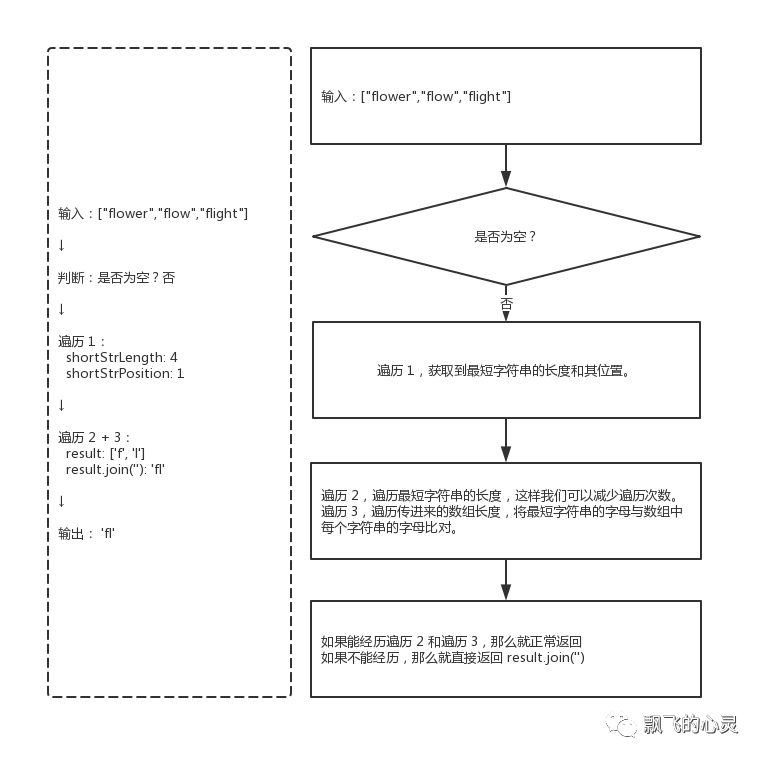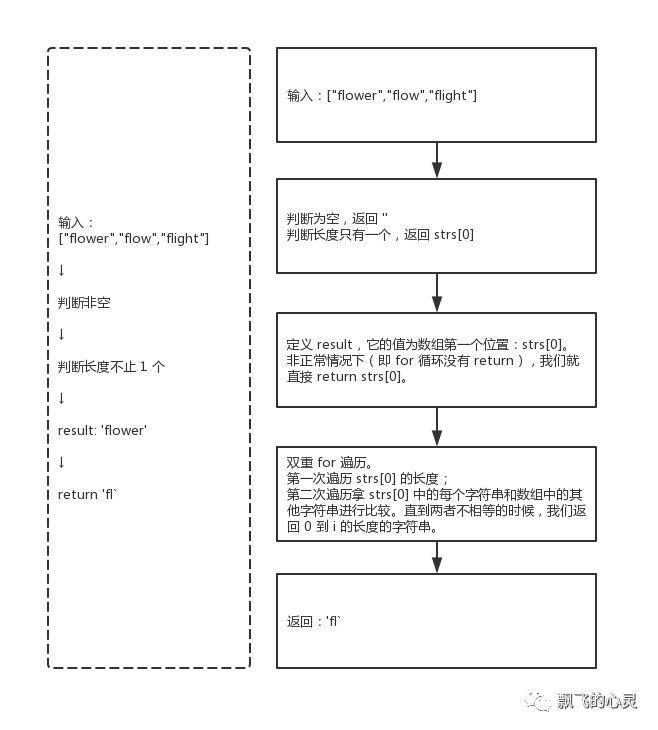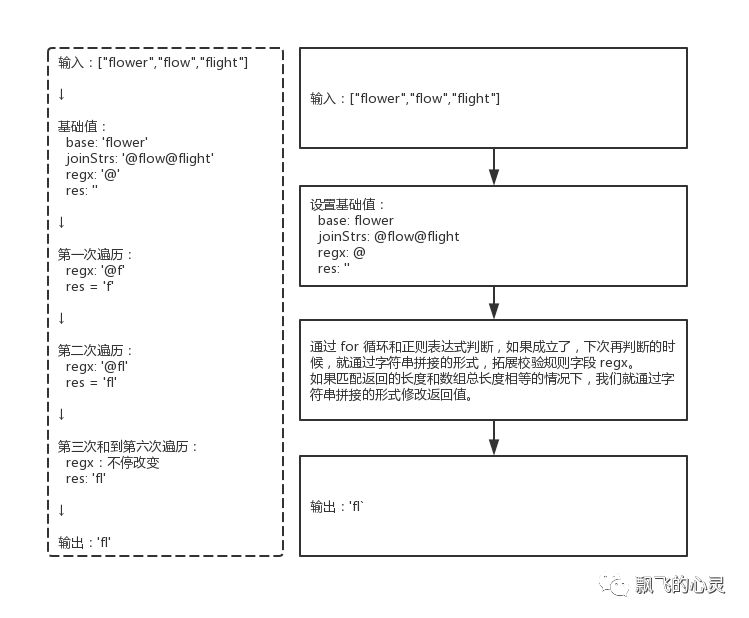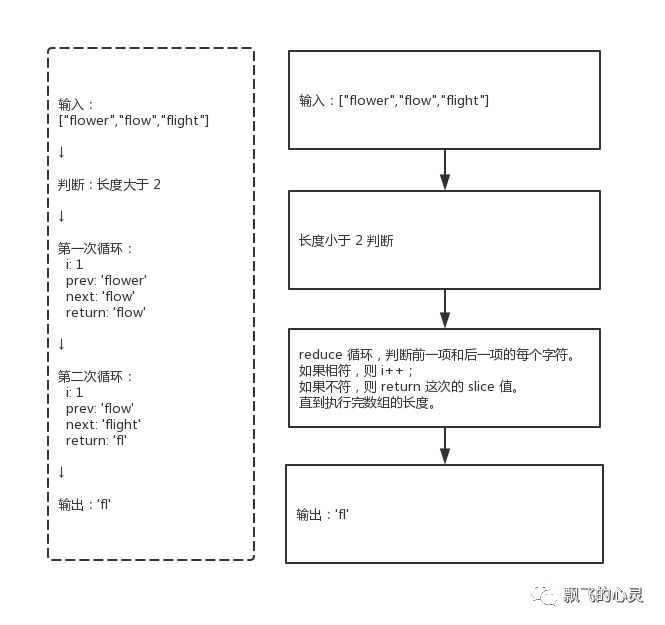## LeetCode - 014 - 最长公共前缀（longest-common-prefix）

jsliang 2019-06-03

Create by jsliang on 2019-06-03 10:13:01
Recently revised in 2019-06-03 17:17:56

## 一 目录

| 目录 | |   ---   | | 一 目录 | | 二 前言 | | 三 解题 | |  3.1 解法 - 暴力破解 | |  3.2 解法 - 水平扫描 | |  3.3 解法 - 正则表达式 | |  3.4 解法 - 水平扫描 |

## 二 前言

• 难度：简单

• 涉及知识：字符串

• 题目地址：https://leetcode-cn.com/problems/longest-common-prefix/

• 题目内容

``编写一个函数来查找字符串数组中的最长公共前缀。``如果不存在公共前缀，返回空字符串 ""。``示例 1:``输入: ["flower","flow","flight"]``输出: "fl"``示例 2:``输入: ["dog","racecar","car"]``输出: ""``解释: 输入不存在公共前缀。``说明:``所有输入只包含小写字母 a-z 。``

## 三 解题

• 官方题解

https://leetcode-cn.com/problems/longest-common-prefix/solution/zui-chang-gong-gong-qian-zhui-by-leetcode/

### 3.1 解法 - 暴力破解

• 解题代码

``var longestCommonPrefix = function(strs) {``  if (!strs.length) {``    return '';``  }``  let shortStrLength = strs.length; // 最短字符串的长度``  let shortStrPosition = 0; // 最短字符串的位置``  for (let i = 0; i < strs.length; i++){``    if (strs[i].length < shortStrLength) {``      shortStrLength = strs[i].length;``      shortStrPosition = i;``    }``  }``  let result = [];``  for (let i = 0; i < shortStrLength; i++) {``    for (let j = 0; j < strs.length; j++) {``      if (strs[shortStrPosition][i] != strs[j][i]) {``        return result.join('');``      }``      if (j === strs.length - 1) {``        result[i] = strs[shortStrPosition][i];``      }``    }``  }``  return result.join('');``};``
• 执行测试 1

1. `strs`： `["flower","flow","flight"]`

2. `return`

``"fl"``
• 执行测试 2

1. `strs`： `["dog","racecar","car"]`

2. `return`

``""``
• LeetCode Submit

``✔ Accepted``  ✔ 118/118 cases passed (92 ms)``  ✔ Your runtime beats 86.97 % of javascript submissions``  ✔ Your memory usage beats 36.33 % of javascript submissions (35.1 MB)``
• 知识点

1. `join()`： `join()` 方法将一个数组（或一个类数组对象）的所有元素连接成一个字符串并返回这个字符串。 `join()` 详细介绍

• 解题思路### 3.2 解法 - 水平扫描

• 解题代码

``var longestCommonPrefix = function(strs) {``  if (strs.length < 2) {``    return !strs.length ? '' : strs;``  }``  var result = strs;``  for(let i = 0; i < result.length; i++) {``    for(let j = 1; j < strs.length; j++) {``      if (result[i] !== strs[j][i]) {``        return result.substring(0, i);``      }``    }``  }``  return result;``};``
• 执行测试 1

1. `strs`： `["flower","flow","flight"]`

2. `return`

``"fl"``
• 执行测试 2

1. `strs`： `["dog","racecar","car"]`

2. `return`

``""``
• LeetCode Submit

``✔ Accepted``  ✔ 118/118 cases passed (88 ms)``  ✔ Your runtime beats 91.9 % of javascript submissions``  ✔ Your memory usage beats 46.38 % of javascript submissions (34.9 MB)``
• 知识点

1. `substring()`： `substring()` 方法将一个数组（或一个类数组对象）的所有元素连接成一个字符串并返回这个字符串。 `substring()` 详细介绍

• 解题思路### 3.3 解法 - 正则表达式

• 解题代码

``var longestCommonPrefix = function(strs) {``  if (strs.length < 2) {``    return !strs.length ? '' : strs;``  }``  let base = strs.shift(),``    joinStrs = '@' + strs.join('@'),``    regx = '@',``    res = '';``  for(let i = 0; i < base.length; i++){``    regx += base.substring(i, i + 1);``    let matchArr = joinStrs.match(new RegExp(`\${regx}`,"g")) || [];``    if(matchArr.length === strs.length){``      res += base.substring(i, i+1);``    }``  }``  return res;``};``
• 执行测试 1

1. `strs`： `["flower","flow","flight"]`

2. `return`

``"fl"``
• 执行测试 2

1. `strs`： `["dog","racecar","car"]`

2. `return`

``""``
• LeetCode Submit

``✔ Accepted``  ✔ 118/118 cases passed (108 ms)``  ✔ Your runtime beats 45.03 % of javascript submissions``  ✔ Your memory usage beats 16.23 % of javascript submissions (35.9 MB)``
• 知识点

1. `join()`： `join()` 方法将一个数组（或一个类数组对象）的所有元素连接成一个字符串并返回这个字符串。 `join()` 详细介绍

2. `substring()`： `substring()` 方法将一个数组（或一个类数组对象）的所有元素连接成一个字符串并返回这个字符串。 `substring()` 详细介绍

3. `RegExp`：构造函数的原型对象。常用语一些便捷操作。 `RegExp` 详细介绍

• 解题思路### 3.4 解法 - 水平扫描

• 解题代码

``var longestCommonPrefix = function(strs) {``  if (strs.length < 2) {``    return !strs.length ? '' : strs;``  }``  return strs.reduce((prev, next) => {``    let i = 0;``    while (prev[i] && next[i] && prev[i] === next[i]) {``      i++;``    };``    return prev.slice(0, i);``  });``};``
• 执行测试 1

1. `strs`： `["flower","flow","flight"]`

2. `return`

``"fl"``
• 执行测试 2

1. `strs`： `["dog","racecar","car"]`

2. `return`

``""``
• LeetCode Submit

``✔ Accepted``  ✔ 118/118 cases passed (80 ms)``  ✔ Your runtime beats 96.32 % of javascript submissions``  ✔ Your memory usage beats 21.06 % of javascript submissions (35.5 MB)``
• 知识点

1. `reduce()`： `reduce()` 方法对数组中的每个元素执行一个由您提供的reducer函数(升序执行)，将其结果汇总为单个返回值。 `reduce()` 详细介绍

2. `slice()`： `slice()` 方法提取一个字符串的一部分，并返回一新的字符串。 `slice()` 详细介绍

• 解题思路jsliang 广告推送：

jsliang 的文档库 由 梁峻荣 采用 知识共享 署名-非商业性使用-相同方式共享 4.0 国际 许可协议进行许可。